# Texas Go Math Grade 2 Lesson 15.2 Answer Key Sort Three-Dimensional Solids

Refer to our Texas Go Math Grade 2 Answer Key Pdf to score good marks in the exams. Test yourself by practicing the problems from Texas Go Math Grade 2 Lesson 15.2 Answer Key Sort Three-Dimensional Solids.

## Texas Go Math Grade 2 Lesson 15.2 Answer Key Sort Three-Dimensional Solids

Explore

Circle the cones. Draw an X on the sphere.HOME CONNECTION • Your child identified the solids on this page to review some of the different kinds of solids.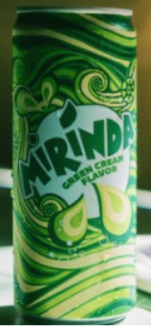Math Talk
Mathematical Processes

The solids in the page are sphere, cylinder, cone, cube, rectangular prism, and triangular prism. They differ in their shapes.

Sphere has no faces, edges, and vertex

The cylinder has 3 faces, 3 edges, and 0 vertex

Cone has 2 faces, 1 edge, and 1 vertex

Cube has 6 faces, 12 edges, and 8 vertices

The rectangular prism has 6 faces, 12 edges, and 8 vertices

The triangular prism has 5 faces, 9 edges, and 6 vertices

Model and Draw

The faces of a cube are squares.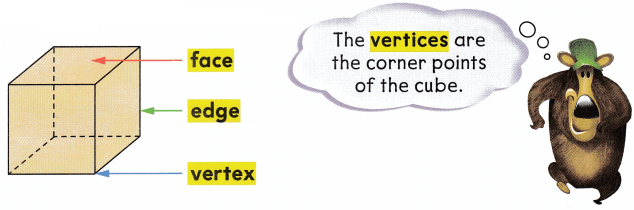Cube has 6 faces, 12 edges, and 8 vertices

Share and Show

Write how many for each.

Question 1.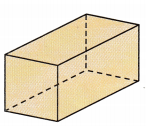rectangular prism
____________ faces
____________ edges
____________ vertices
rectangular prism
______6____ faces
______12___ edges
______8___ vertices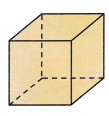cube
____________ faces
____________ edges
____________ vertices
cube
______6______ faces
______12_____ edges
_____8_______ vertices

Cubes are a special kind of rectangular prism. Talk about why this is true.
The cubes are a special kind of rectangular prisms. Yes, it’s true. The cubes are a different kind of rectangular prism as they have the same number of faces, edges, and vertices. There is a small difference in that the cube has equal size throughout whereas rectangular prisms have different sizes of faces.

The length and breadth of the cube are equal on all sides, whereas they differ for the rectangular prism.

Problem Solving

Write how many for each.

Question 3.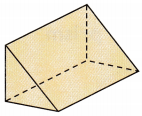triangular prism
____________ faces
____________ edges
____________ vertices
triangular prism
____5______ faces
_____9_____edges
______6____ vertices

Solve. Write or draw to explain.

Question 4.
H.O.T. Multi-Step Two of the six faces of a solid are shown. Draw the rest of the faces.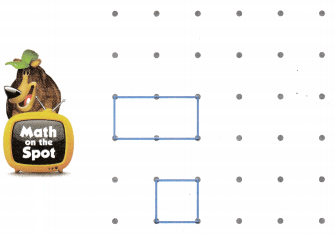What is the name of the solid?
Rectangular prism

Explanation:
The faces shown above belong to a rectangular prism.Question 5.
H.O.T. Look at the faces of the cube and the faces of the rectangular prism. Are they different? Explain.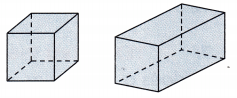Yes

Explanation:
Though the number of faces on the cube and rectangular prisms are same. The sizes of the faces i.e, the length and breadth of the rectangular prism differ from the cube.

Write the shape names to complete.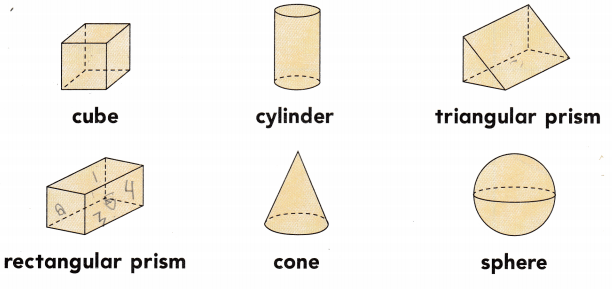Decide which labels are true for each solid. Then write the names of those solids.
Has exactly 6 faces

Has exactly 6 vertices

Has exactly 12 edges
Cube and Rectangular prism has 6 faces.
A triangular prism has 6 vertices.
Cube, rectangular prism, pentagonal prism, and Hexagonal pyramid have 12 edges.

Question 7.
Texas Test Prep How many faces does a triangular prism have?(A) 5
(B) 9
(C) 6
5

TAKE HOME ACTIVITY • Have your child tell you about the faces on a cereal box or another kind of box.
6
Explanation:
The cereal box or any kind of box consists of 6 faces, irrespective of size and shape.

Texas Go Math Grade 2 Lesson 15.2 Homework and Practice Answer Key

Write how many for each.

Question 1.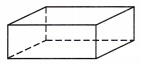rectangular prism
____________ faces
____________ edges
____________ vertices
rectangular prism
______6____ faces
______12___ edges
______8___ vertices

Question 2.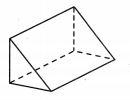triangular prism
____________ faces
____________ edges
____________ vertices
____5______ faces
____9_____edges
_____6___ vertices

Problem Solving

Question 3.
Multi-Step Three of the six faces of a solid are shown. Draw the rest of the faces.What is the name of the solid?
Cube

Explanation:
As the three faces of the solid are of the same size, the solid is a cube.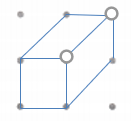Lesson Check

Question 4.
How many faces does a triangular prism have?(A) 9
(B) 6
(C) 5
5 faces

Question 5.
Gina’s cereal box has 6 faces, 12 edges, and 8 vertices. What is the name of the shape of her cereal box?
(A) cylinder
(B) rectangular prism
(C) triangular prism
Rectangular prism

Dave gives his brother a toy car in a box that is a rectangular prism with 6 square faces. Which is another name for this rectangular prism?
(A) cube
(B) cone
(C) triangular prism